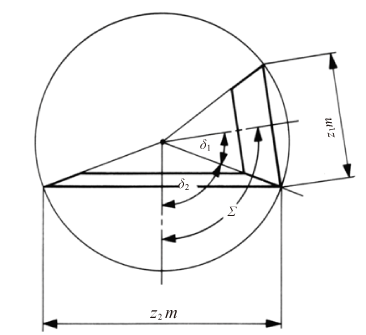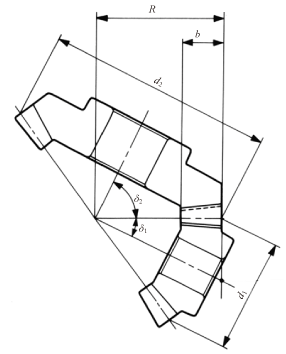Home  •  Tutorials  •  Calculators  •  3D Models  •  About  •  Contact

Bevel Gear Calculator

The following online calculator computes the basic dimensions and tooth profiles of a bevel gear pair (pinion and gear) based on their number of teeth and angle between the shaft axes.

Conceptually, two meshing bevel gears can be represented by two touching cones, referred to as pitch cones or reference cones, shown in thick lines on the left image below.

The diameter of a cone's base circle is simply the gear's number of teeth times module (z1m and z2m). The angle between the shaft axes, Σ, is usually 90° but could be a different number. Finally, the facewidth b is the length of the contact line of the two reference cones (see right image). This value should not exceed 1/3 of the Cone distance denoted R on the right image, which is also the radius of the circle shown on the left image.

This calculator takes m, z1, z2, Σ and b as inputs, and computes the parametric equations for the tooth profiles of both gears, the cone angles δ1 and δ2, cone distance R, a scaling factor s calculated as (R - b) / R, and distance from the cone base to apex. The scaling factor makes it easy to model the top section of the gear by scaling down its bottom section, and the base-to-apex height is used to correctly position the gear's origin, as shown in the tutorial.

Update: As of 2017-01-18, this calculator is equipped with two new buttons, Show pinion script and Show gear script. These buttons generate Python scripts that instantly tranform flat gear outlines to saucer-like shapes that form the top and bottom of a bevel gear.Basic Dimensions
ParameterSymbolPinionGear
Modulem
Number of teethz
Shaft angle (°)Σ
Facewidthb
Reference radius (mz / 2)r
Reference cone angle (°)δ
Cone distanceR
Cone base-to-apex height (R cos δ)h
Top/Bottom Scaling Factors
Transformation Python Script
Tooth thickness at base (°)ψb

 X equation Y equation Z equation Umin, Umax, Ustep

 X equation Y equation Z equation Umin, Umax, Ustep